# Compose 中的狀態簡介

## 1. 事前須知

• 無法建立網路連線時顯示的訊息。
• 表單，例如註冊表單。狀態可能是「已填寫」和「已提交」。
• 觸控式控制項，例如按鈕。狀態可能是「未輕觸」、「輕觸中」(顯示動畫) 或「已輕觸」(`onClick` 動作)。

• 用於輸入和編輯文字的 `TextField` 可組合項。
• 用於顯示文字的 `Text` 可組合項。
• 用於在 UI 元素之間顯示空白空間的 `Spacer` 可組合項。## 必要條件

• 瞭解 Compose 的基本知識，例如 `@Composable` 註解。
• 熟悉 Compose 版面配置的基本知識，例如 `Row``Column` 版面配置可組合項。
• 熟悉修飾符的基本知識，例如 `Modifier.padding()` 函式。
• 熟悉 `Text` 可組合項。

## 課程內容

• 如何考量 UI 中的狀態。
• Compose 如何使用狀態顯示資料。
• 如何在應用程式中加入文字方塊。
• 如何提升狀態。

## 建構項目

• 一款小費計算機應用程式 Tip Time，可根據服務金額計算小費金額。

## 軟硬體需求

• 一台可連上網際網路並具備網路瀏覽器的電腦
• Kotlin 知識
• Android Studio

## 3. 開始操作

1. 請查看 Google 線上小費計算機。請注意，這只是範例，並非您稍後要在本課程中建立的 Android 應用程式。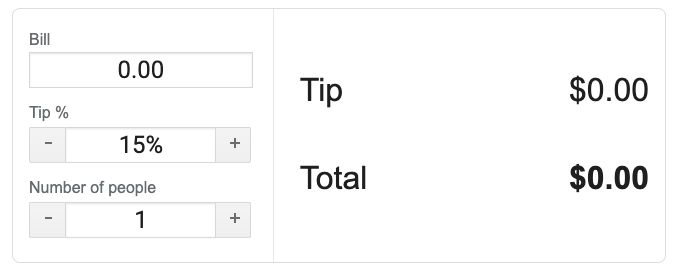1. 在「帳單」和「小費」方塊中輸入不同的值。小費和總金額也會隨之改變。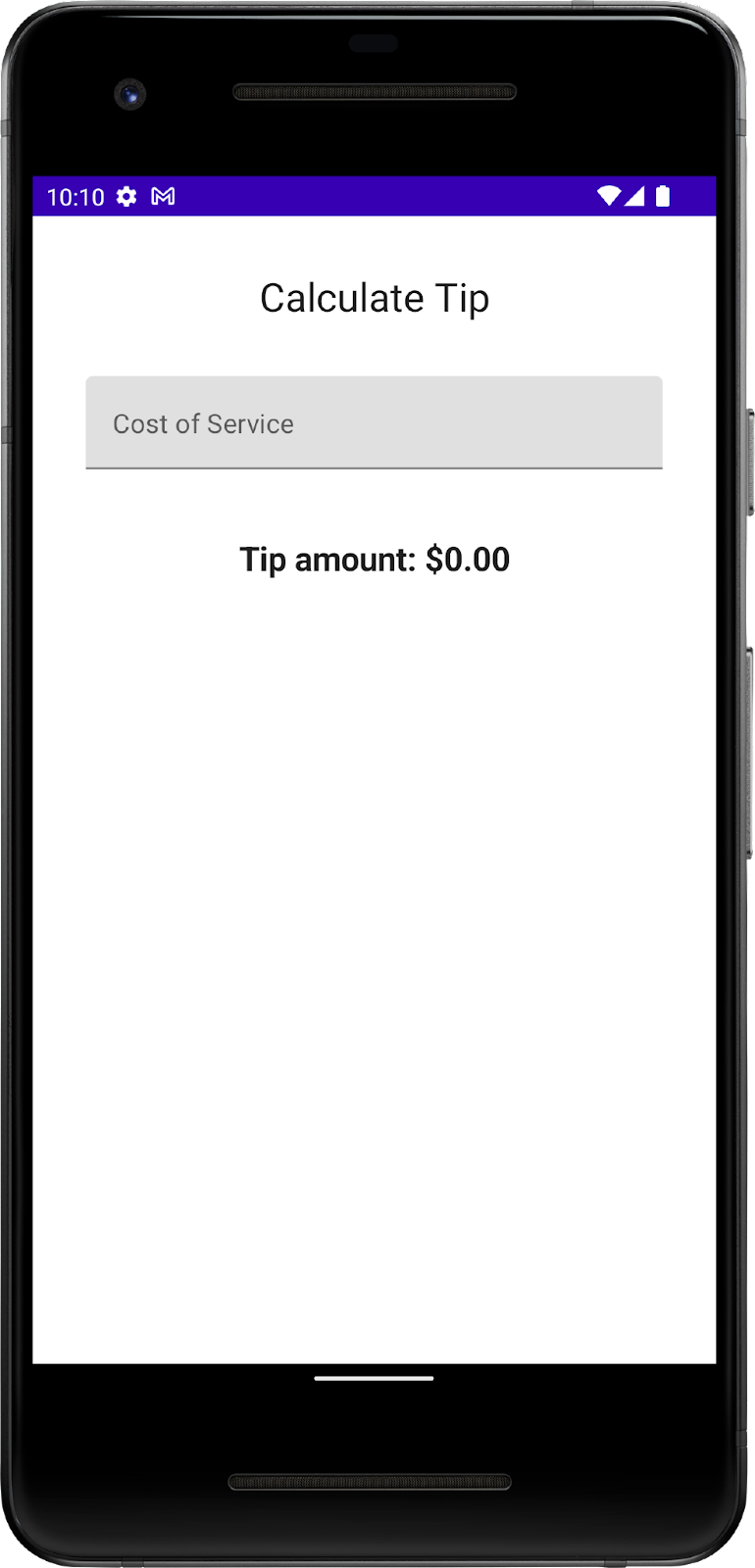## 4. 建立專案

1. 在 Android Studio 中，使用「Empty Compose Activity」(空白 Compose 活動) 範本建立專案，然後輸入 `Tip Time` 做為專案名稱，接著選取「API 21: Android 5.0 (Lollipop)」以上版本做為最低 SDK。載入專案檔案。
2. 在「Project」(專案) 窗格中，按一下「res > values > string.xml」。您應該為應用程式名稱設定單一字串資源。
3. `<resources>` 標記之間，輸入下列字串資源：
``````<string name="calculate_tip">Calculate Tip</string>
<string name="cost_of_service">Cost of Service</string>
<string name="tip_amount">Tip amount: %s</string>
``````

`strings.xml` 檔案應該如以下程式碼片段所示：

`strings.xml`

``````<resources>
<string name="app_name">Tip Time</string>
<string name="calculate_tip">Calculate Tip</string>
<string name="cost_of_service">Cost of Service</string>
<string name="tip_amount">Tip amount: %s</string>
</resources>
``````

## 5. 新增畫面標題

1. `MainActivity.kt` 檔案中，刪除 `Greeting()` 函式：
``````// Delete this.
@Composable
fun Greeting(name: String) {
//...
}
``````
1. `onCreate()``DefaultPreview()` 函式中，刪除 `Greeting()` 函式呼叫：
``````// Delete this.
Greeting("Android")
``````
1. `onCreate()` 函式下方，新增 `TipTimeScreen()` 可組合函式來表示應用程式畫面：
``````@Composable
fun TipTimeScreen() {
}
``````
1. `onCreate()` 函式的 `Surface()` 區塊中，呼叫 `TipTimeScreen()` 函式：
``````override fun onCreate(savedInstanceState: Bundle?) {
//...
setContent {
TipTimeTheme {
Surface(
//...
) {
TipTimeScreen()
}
}
}
}
``````
1. `DefaultPreview()` 函式的 `TipTimeTheme` 區塊中，呼叫 `TipTimeScreen()` 函式：
``````@Preview(showBackground = true)
@Composable
fun DefaultPreview() {
TipTimeTheme {
TipTimeScreen()
}
}
``````

## 顯示畫面標題

1. `TipTimeScreen()` 函式中，新增 `Column` 元素。這些元素位於垂直欄中，因此您必須使用 `Column` 元素。
2. `Column` 區塊中，將 `modifier` 命名參數設為用於接受 `32.dp` 引數的 `Modifier.padding` 函式：
``````Column(
) {}
``````
1. 匯入以下函式以及這個屬性：
``````import androidx.compose.foundation.layout.Column
import androidx.compose.ui.unit.dp
``````
1. `Column` 函式中，傳入 `verticalArrangement` 命名引數並將其設為可接受 `8.dp` 引數的 `Arrangement.spacedBy` 函式：
``````Column(
verticalArrangement = Arrangement.spacedBy(8.dp)
) {}
``````

1. 匯入以下函式：
``````import androidx.compose.foundation.layout.Arrangement
``````
1. 新增 `Text` 元素，讓該元素接受設為 `stringResource(R.string.``calculate_tip``)` 函式的 `text` 命名參數、設為 `24.sp` 值的 `fontSize` 命名參數，以及設為 `Modifier.align(Alignment.CenterHorizontally)` 函式的 `modifier` 命名引數：
``````Text(
text = stringResource(R.string.calculate_tip),
fontSize = 24.sp,
modifier = Modifier.align(Alignment.CenterHorizontally)
)
``````
1. 匯入以下匯入項目：
``````import androidx.compose.ui.res.stringResource
import androidx.compose.ui.unit.sp
import androidx.compose.ui.Alignment
``````
1. 在「設計」窗格中，按一下「Create & Refresh」(建立並重新整理)。畫面上應該會顯示 `Calculate Tip` 這個畫面標題，也就是您新增的文字元素。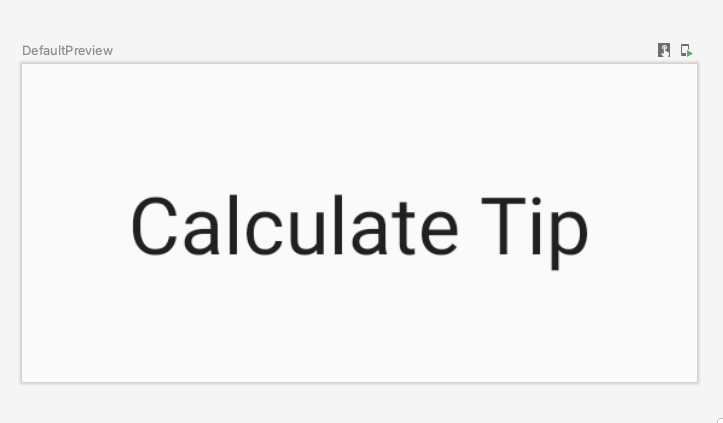## 新增 `TextField`可組合項`TextField` 可組合函式可讓使用者在應用程式內輸入文字。例如，請注意以下圖片中 Gmail 應用程式登入畫面的文字方塊：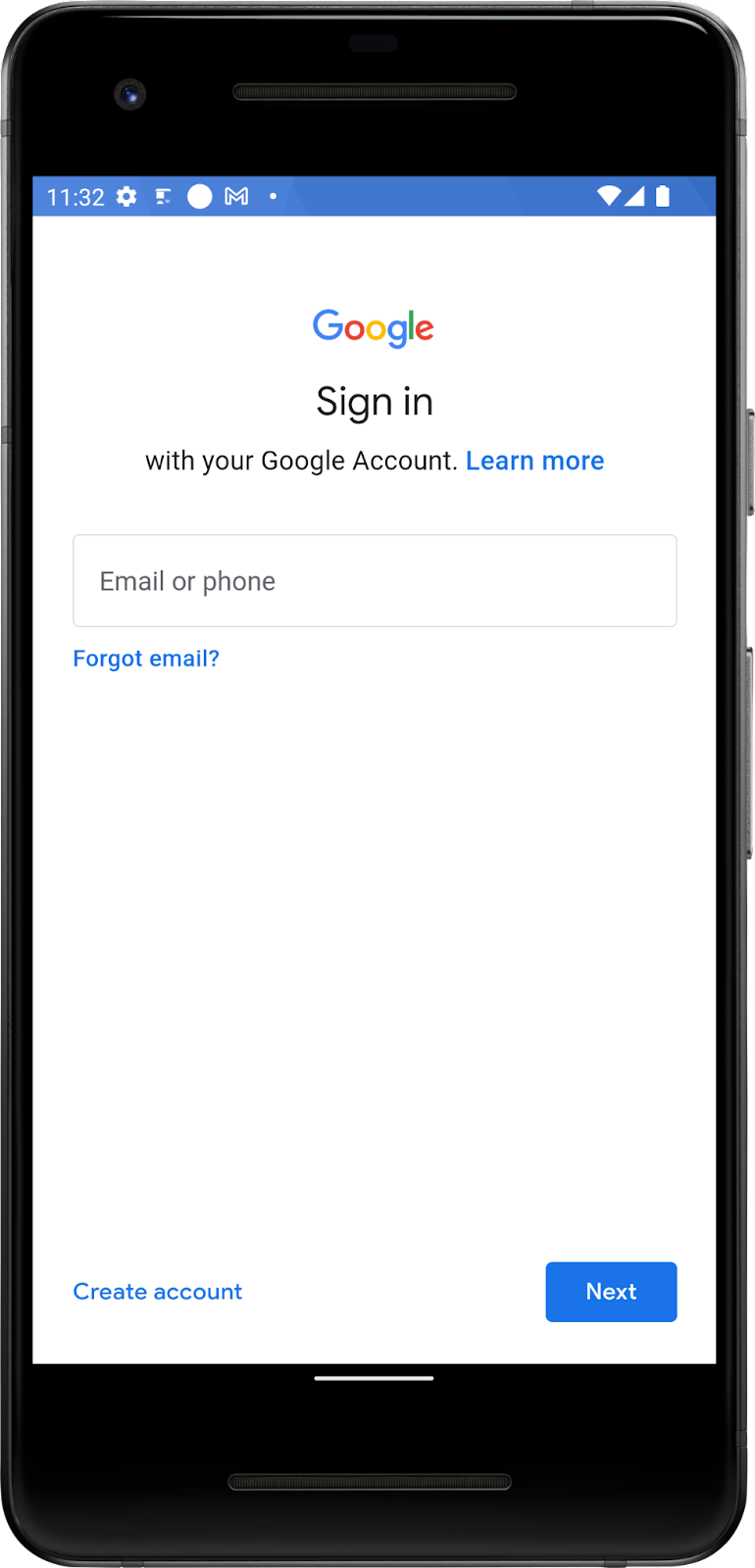1. `Text` 元素後方的 `Column` 區塊中，新增 `Spacer()` 可組合函式 (高度為 `16dp`)。
``````@Composable
fun TipTimeScreen() {
Column(
verticalArrangement = Arrangement.spacedBy(8.dp)
) {
Text(
...
)
Spacer(Modifier.height(16.dp))
}
}
``````

1. 匯入以下函式：
``````import androidx.compose.foundation.layout.Spacer
import androidx.compose.foundation.layout.height
``````
1. `MainActivity.kt` 檔案中，新增 `EditNumberField()` 可組合函式。
2. `EditNumberField()` 函式的內文中新增 `TextField`，用於接受設為空字串的 `value` 命名參數，以及設為空白 lambda 運算式的 `onValueChange` 命名參數：
``````@Composable
fun EditNumberField() {
TextField(
value = "",
onValueChange = {},
)
}
``````
1. 請注意您傳遞的參數：
• `value` 參數是文字方塊，其中會顯示您在這裡傳遞的字串值。
• `onValueChange` 參數是使用者在文字方塊中輸入文字時觸發的 lambda 回呼。
1. 匯入這個函式：
``````import androidx.compose.material.TextField
``````
1. `Spacer()` 可組合函式後方的行中，呼叫 `EditNumberField()` 函式：
``````@Composable
fun TipTimeScreen() {
Column(
verticalArrangement = Arrangement.spacedBy(8.dp)
) {
Text(
...
)
Spacer(Modifier.height(16.dp))
EditNumberField()
}
}
``````

1. 在「設計」窗格中，按一下「Create & Refresh」(建立並重新整理) 圖示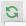。您應該會看到 `Calculate Tip` 這個畫面標題以及一個包含 `16dp` 空間的空白文字方塊。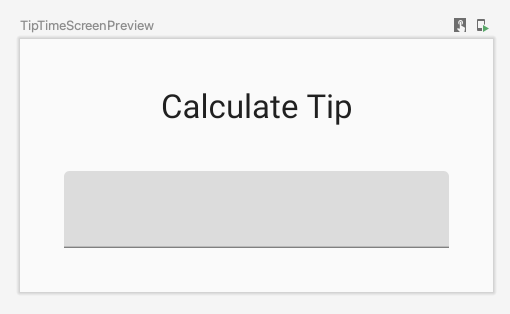## 6. 使用 Compose 中的狀態

1. `EditNumberField()` 函式的開頭，使用 `val` 關鍵字新增指派給靜態值 `"0"``amountInput` 變數：
``````val amountInput = "0"
``````

1. `value` 命名參數設為 `amountInput` 值：
``````TextField(
value = amountInput,
onValueChange = {},
)
``````
1. 再次建構並執行應用程式。文字方塊會顯示設為狀態變數的值，如下圖所示：1. 輸入其他值。由於 `TextField` 可組合項不會自行更新，硬式編碼狀態仍維持不變。當 `value` 參數變更 (設為 `amountInput` 屬性) 時，這個可組合項會隨之更新。

`amountInput` 變數代表文字方塊的狀態。經過硬式編碼的狀態無法修改，也不會反映使用者輸入內容，因此沒有實用性。當使用者更新服務費時，您必須更新應用程式的狀態。

## 7. 組成

Compose 是宣告式 UI 架構，代表您可以透過程式碼「宣告」 UI 的外觀。如果您想讓文字方塊一開始就顯示 `100` 值，請在程式碼中將可組合函式的初始值設為 `100` 值。

「組成」是指 Compose 在執行可組合項時建構的 UI 描述。Compose 應用程式會呼叫可組合函式，將資料轉換為 UI。如果狀態變更，Compose 會以新的狀態重新執行受影響的可組合函式，進而建立新的 UI，這個過程稱為「重新組成」。Compose 會為您建立「重新組成」排程。

`mutableStateOf()` 函式傳回的值：

• 保留狀態，也就是服務費。
• 可變動，也就是可以變更這個值。
• 可觀察，也就是 Compose 會觀察這個值發生的任何變更，並觸發重新組成以更新 UI。

1. `EditNumberField()` 函式中，將 `amountInput` 狀態變數之前的 `val` 關鍵字變更為 `var` 關鍵字：
``````var amountInput = "0"
``````

1. 使用 `MutableState<String>` 類型 (而非經過硬式編碼的 `String` 變數)，讓 Compose 知道要追蹤 `amountInput` 狀態並傳入 `"0"` 字串，也就是 `amountInput` 狀態變數的初始預設值：
``````var amountInput: MutableState<String> = mutableStateOf("0")
``````

`amountInput` 初始化程序也可以類型推論的方式編寫，如下所示：

``````var amountInput = mutableStateOf("0")
``````

`mutableStateOf()` 函式會接受做為參數封裝在 `State` 物件中的初始值 `"0"`，使其 `value` 變為可觀察狀態。這會導致 Android Studio 發出以下編譯警告，但您很快可以加以修正：

``````Creating a state object during composition without using remember.
``````
1. `TextField` 可組合函式中，使用 `amountInput.value` 屬性：
``````TextField(
value = amountInput.value,
onValueChange = { },
)
``````

Compose 會追蹤每個可讀取狀態 `value` 屬性的可組合項，並在其 `value` 變更時觸發重新組成。

1. `onValueChange` 命名參數的 lambda 運算式中，將 `amountInput.value` 屬性設為 `it` 變數：
``````@Composable
fun EditNumberField() {
var amountInput = mutableStateOf("0")
TextField(
value = amountInput.value,
onValueChange = { amountInput.value = it },
)
}
``````

`TextField` 透過 `onValueChange` 回呼函式通知您文本發生變更時，您將更新`TextField` 狀態 (也就是 `amountInput` 變數)。

1. 執行應用程式，並在文字方塊中輸入文字。如下圖所示，文字方塊仍然會顯示 `0` 值：## 8. 使用「記住」功能儲存狀態

`EditNumberField()` 函式中，使用 `remember` 函式：

1. `EditNumberField()` 函式中，使用 `remember` 括住 `mutableStateOf``()` 函式呼叫，以便透過 `by` `remember` Kotlin 資源委派初始化 `amountInput` 變數。
2. `mutableStateOf``()` 函式中，傳入空字串 (而非靜態 `"0"` 字串)：
``````var amountInput by remember { mutableStateOf("") }
``````

1. 匯入以下函式：
``````import androidx.compose.runtime.mutableStateOf
import androidx.compose.runtime.remember
``````
1. 手動匯入以下函式：
``````import androidx.compose.runtime.getValue
import androidx.compose.runtime.setValue
``````

``````@Composable
fun EditNumberField() {
var amountInput by remember { mutableStateOf("") }
TextField(
value = amountInput,
onValueChange = { amountInput = it },
)
}
``````
1. 執行應用程式，並在文字方塊中輸入一些文字。您現在應該可以看到先前輸入的文字。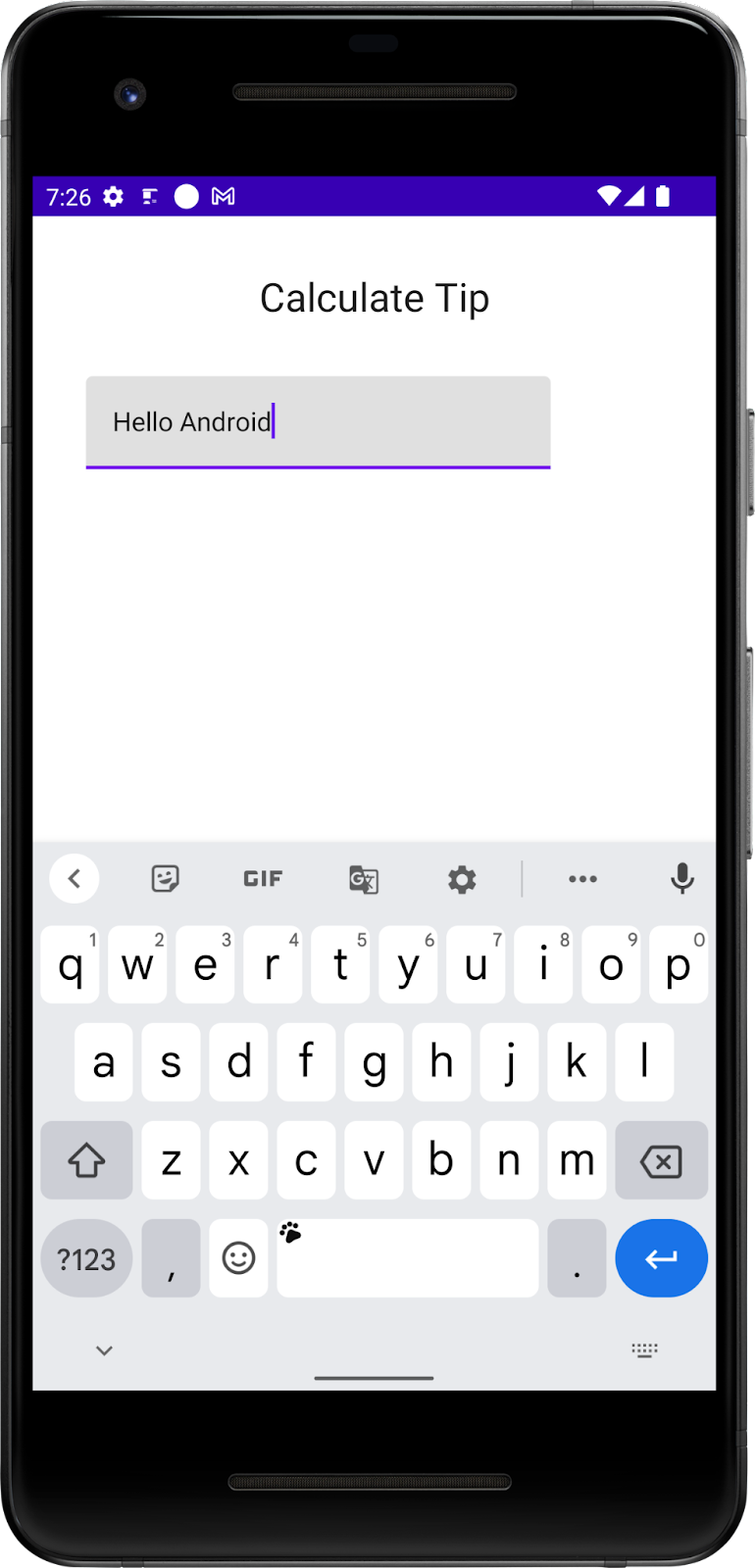## 9. 狀態與重新組成實際使用教學

1. `onValueChange` 命名參數旁邊的 `EditNumberField()` 函式中，設定行中斷點。
2. 在導覽選單中，按一下「Debug 'app'」(對應用程式進行偵錯)。應用程式會在模擬器或裝置上啟動。建立 `TextField` 元素後，應用程式的執行程序將首次暫停。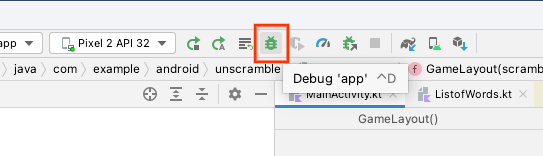1. 在「Debug」(偵錯) 窗格中，按一下「Continue Program」(繼續執行程式) 圖示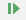。文字方塊建立完成。
2. 在模擬器或裝置的文字方塊中輸入字母。到達您設定的中斷點時，應用程式就會再次暫停執行程序。1. 在「Debug」(偵錯) 窗格中，按一下「Continue Program」(繼續執行程式) 圖示。在模擬器或裝置上輸入的文字會顯示在包含中斷點的程式碼行旁，如下圖所示：1. 按一下「Resume Program」圖示。您輸入的值會顯示在模擬器或裝置上。

## 在文字方塊中新增標籤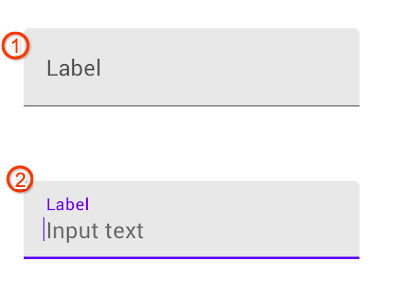1. `EditNumberField()` 函式的 `TextField()` 可組合函式中，新增一個設為空白 lambda 運算式的 `label` 命名參數：
``````TextField(
//...
label = { }
)
``````
1. 在 lambda 運算式中，呼叫用於接受 `stringResource``(R.string.``cost_of_service``)``Text()` 函式：
``````label = { Text(stringResource(R.string.cost_of_service)) }
``````
1. `TextField()` 可組合函式中，新增 `modifier` 命名參數並將值設為 `Modifier.``fillMaxWidth``()`
``````TextField(
// Other parameters
modifier = Modifier.fillMaxWidth(),
)
``````
1. 匯入下列項目：
``````import androidx.compose.foundation.layout.fillMaxWidth
``````
1. `TextField()` 可組合函式中，新增 `singleLine` 命名參數並將值設為 `true`
``````TextField(
// Other parameters
singleLine = true,
)
``````

1. 新增 `keyboardOptions` 命名參數，並設為 `KeyboardOptions()`
``````TextField(
// Other parameters
keyboardOptions = KeyboardOptions()
)
``````

Android 提供選項讓您設定螢幕上顯示的鍵盤，以便輸入數字、電子郵件地址、網址和密碼等內容。如要進一步瞭解其他鍵盤類型，請參閱 KeyboardType

1. 將鍵盤類型設為數字鍵盤，即可輸入數字。為 `KeyboardOptions` 函式傳遞 `KeyboardType.Number` 命名參數並將值設為 `keyboardType`
``````TextField(
// Other parameters
keyboardOptions = KeyboardOptions(keyboardType = KeyboardType.Number)
)
``````

``````@Composable
fun EditNumberField() {
var amountInput by remember { mutableStateOf("") }
TextField(
value = amountInput,
onValueChange = { amountInput = it },
label = { Text(stringResource(R.string.cost_of_service)) },
modifier = Modifier.fillMaxWidth(),
singleLine = true,
keyboardOptions = KeyboardOptions(keyboardType = KeyboardType.Number)

)
}
``````
1. 匯入下列項目：
``````import androidx.compose.ui.text.input.KeyboardType
import androidx.compose.foundation.text.KeyboardOptions
``````
1. 執行應用程式。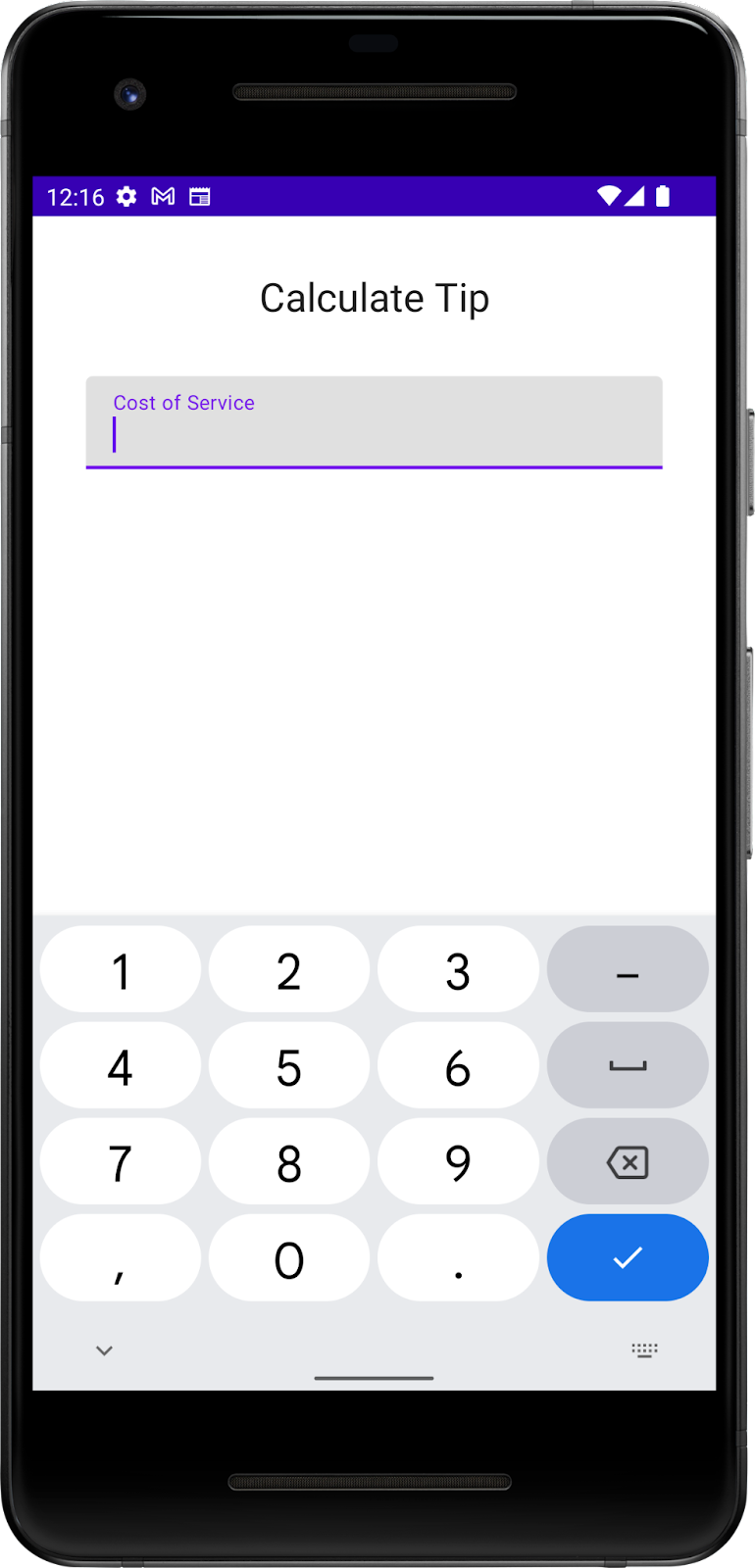## 11. 顯示小費金額## 計算小費金額

1. `EditNumberField()` 函式後方的 `MainActivity.kt` 檔案中，新增 `private` `calculateTip()` 函式。
2. 新增 `amount``tipPercent` 命名參數，兩者皆為 `Double` 類型。`amount` 參數會傳遞服務費。
3. `tipPercent` 參數設為 `15.0` 預設引數值。這會將預設的小費百分比設為 15%。在下一個程式碼研究室中，您將取得使用者提供的消費金額：
``````private fun calculateTip(
amount: Double,
tipPercent: Double = 15.0
) {
}
``````
1. 在函式內文中，使用 `val` 關鍵字定義 `tip` 變數，將 `tipPercent` 參數除以 `100` 值，然後將結果乘以 `amount` 參數即可計算小費：
``````private fun calculateTip(
amount: Double,
tipPercent: Double = 15.0
) {
val tip = tipPercent / 100 * amount
}
``````

1. `calculateTip()` 函式內文的下一行中，呼叫 `NumberFormat.getCurrencyInstance()` 函式：
``````NumberFormat.getCurrencyInstance()
``````

1. 呼叫 `NumberFormat.getCurrencyInstance()` 函式時，鏈結 `format()` 方法並將 `tip` 變數做為參數傳入：
``````NumberFormat.getCurrencyInstance().format(tip)
``````
1. 當 Android Studio 發出提示時，匯入這個類別。
``````import java.text.NumberFormat
``````
1. 最後一個步驟是，從函式傳回格式化字串。修改函式簽名以傳回 `String` 類型。在 `NumberFormat` 陳述式前面新增 `return` 關鍵字：
``````private fun calculateTip(
amount: Double,
tipPercent: Double = 15.0
): String {
val tip = tipPercent / 100 * amount
return NumberFormat.getCurrencyInstance().format(tip)
}
``````

## 使用 `calculateTip()` 函式

1. `EditNumberField()` 可組合函式中，呼叫 `amountInput` 變數中的 `toDoubleOrNull` 函式，以便將 `String` 轉換為 `Double`
``````val amount = amountInput.toDoubleOrNull()
``````

`toDoubleOrNull()` 函式是預先定義的 Kotlin 函式，可將字串分析為 `Double` 數字並傳回結果；如果字串並不是有效數值，則傳回 `null`

1. 請在陳述式結尾新增 `?:` Elvis 運算子，以便在 `amountInput` 為空值時傳回 `0.0` 值：
``````val amount = amountInput.toDoubleOrNull() ?: 0.0
``````
1. `amount` 變數後，建立另一個名為 `tip``val` 變數。使用 `calculateTip()` 初始化這個變數，並傳遞 `amount` 參數。
``````val tip = calculateTip(amount)
``````

`EditNumberField()` 函式應如下列程式碼片段所示：

``````@Composable
fun EditNumberField() {
var amountInput by remember { mutableStateOf("") }

val amount = amountInput.toDoubleOrNull() ?: 0.0
val tip = calculateTip(amount)

TextField(
value = amountInput,
onValueChange = { amountInput = it },
label = { Text(stringResource(R.string.cost_of_service)) },
modifier = Modifier.fillMaxWidth(),
singleLine = true,
keyboardOptions = KeyboardOptions(keyboardType = KeyboardType.Number)
)
}
``````

## 顯示小費金額計算結果1. `Column()` 區塊結尾的 `TipTimeScreen()` 函式中，新增 `Spacer()` 可組合項並傳入粗細值 `24.dp`
``````@Composable
fun TipTimeScreen() {
Column(
//...
) {
Text(
//...
)
//...
EditNumberField()
Spacer(Modifier.height(24.dp))
}
}
``````

1. `Spacer()` 可組合項之後，新增下列 `Text` 可組合項：
``````Text(
text = stringResource(R.string.tip_amount, ""),
modifier = Modifier.align(Alignment.CenterHorizontally),
fontSize = 20.sp,
fontWeight = FontWeight.Bold
)
``````

1. 匯入以下匯入項目：
``````import androidx.compose.ui.text.font.FontWeight
``````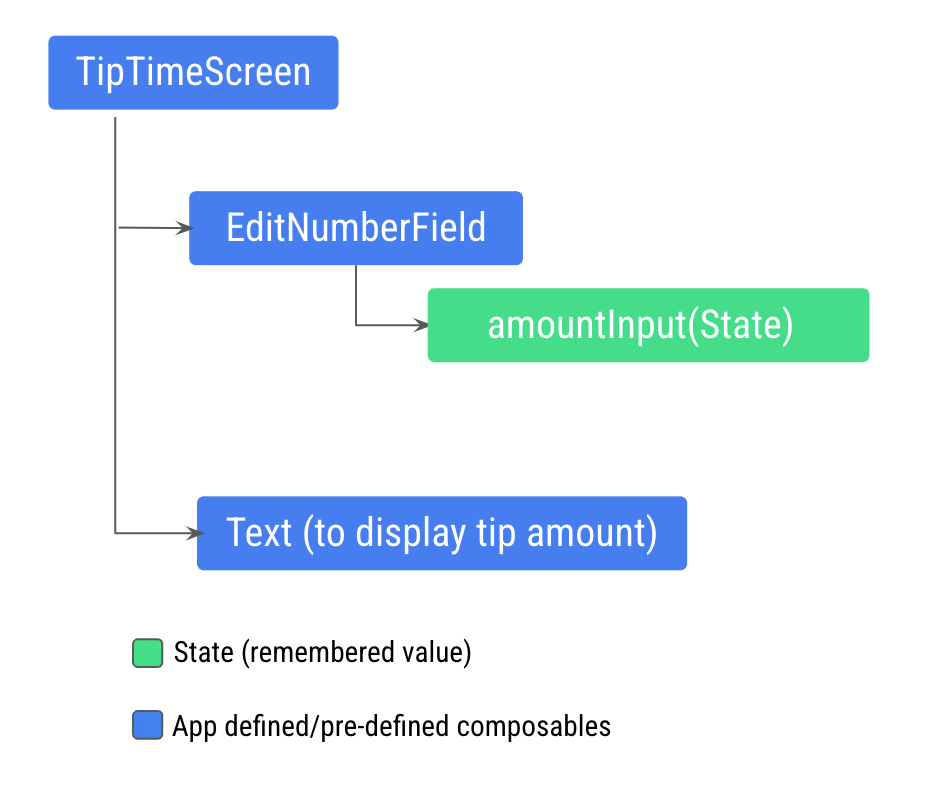## 瞭解有狀態與無狀態的可組合項

• 使用多個可組合函式分享狀態。
• 建立可以在應用程式中重複使用的無狀態可組合項。

「無狀態」可組合函式不具有任何狀態，這表示它不會保留、定義或修改新狀態。另一方面，「有狀態」可組合函式則具有某種可隨時間變更的狀態。

「狀態提升」是指將狀態提升到其他函式的一種機制，目的是讓特定的元件處於無狀態。

• `value: T` 參數，這是要顯示的現值。
• `onValueChange: (T) -> Unit` - 回呼 lambda，在值發生變更時就會觸發，以便在其他位置時更新狀態 (例如使用者在文字方塊中輸入文字時)。

1. 更新 `EditNumberField()` 函式定義，以便透過新增 `value``onValueChange` 參數來提升狀態：
``````fun EditNumberField(
value: String,
onValueChange: (String) -> Unit
)
``````

`value` 參數的類型是 `String`，而 `onValueChange` 參數的類型則是 `(String) -> Unit`，因此這個函式可以接受 `String` 值做為輸入，且沒有傳回值。`onValueChange` 參數用於當做 `onValueChange` 回呼傳遞至 `TextField` 可組合項。

1. `EditNumberField()` 函式中，更新 `TextField()` 可組合函式以使用傳入的參數：
``````TextField(
value = value,
onValueChange = onValueChange,
// Rest of the code
)
``````
1. 將所記住的狀態從 `EditNumberField()` 函式移至 `TipTimeScreen()` 函式，以便提升狀態：
``````@Composable
fun TipTimeScreen() {
var amountInput by remember { mutableStateOf("") }

val amount = amountInput.toDoubleOrNull() ?: 0.0
val tip = calculateTip(amount)

Column(
//...
) {
//...
}
}
``````
1. 您已將狀態提升至 `TipTimeScreen()`，現在請將其傳遞至 `EditNumberField()`。在 `TipTimeScreen()` 函式中，將 `EditNumberField``()` 函式呼叫更新為使用提升後的狀態：
``````EditNumberField(value = amountInput,
onValueChange = { amountInput = it }
)
``````
1. 使用 `tip` 屬性顯示小費金額。更新 `Text` 可組合項的 `text` 參數，以便將 `tip` 變數用做參數。這就是位置格式設定
``````Text(
text = stringResource(R.string.tip_amount, tip),
// Rest of the code
)
``````

``````// No need to copy.

// In the res/values/strings.xml file
<string name="tip_amount">Tip Amount: %s</string>

Text(
text = stringResource(R.string.tip_amount, tip)
)
``````

``````@Composable
fun TipTimeScreen() {
var amountInput by remember { mutableStateOf("") }

val amount = amountInput.toDoubleOrNull() ?: 0.0
val tip = calculateTip(amount)

Column(
verticalArrangement = Arrangement.spacedBy(8.dp)
) {
Text(
text = stringResource(R.string.calculate_tip),
fontSize = 24.sp,
modifier = Modifier.align(Alignment.CenterHorizontally)
)
Spacer(Modifier.height(16.dp))
EditNumberField(value = amountInput,
onValueChange = { amountInput = it }
)
Spacer(Modifier.height(24.dp))
Text(
text = stringResource(R.string.tip_amount, tip),
modifier = Modifier.align(Alignment.CenterHorizontally),
fontSize = 20.sp,
fontWeight = FontWeight.Bold
)
}

}

@Composable
fun EditNumberField(
value: String,
onValueChange: (String) -> Unit
) {
TextField(
value = value,
onValueChange = onValueChange,
label = { Text(stringResource(R.string.cost_of_service)) },
modifier = Modifier.fillMaxWidth(),
singleLine = true,
keyboardOptions = KeyboardOptions(keyboardType = KeyboardType.Number)
)
}
``````

1. 在模擬器或裝置上執行應用程式，然後在「Cost of Service」文字方塊中輸入值。系統隨即會顯示 15% 的小費金額，如下圖所示：## 13. 取得解決方案程式碼

```\$ git clone https://github.com/google-developer-training/basic-android-kotlin-compose-training-tip-calculator.git
\$ cd basic-android-kotlin-compose-training-tip-calculator
\$ git checkout state
```

## 摘要

• 應用程式中的狀態指任何可能隨時間變化的值。
• 「組成」是指 Compose 在執行可組合項時建構的 UI 描述。Compose 應用程式會呼叫可組合函式，將資料轉換為 UI。
• 初始組成是指 Compose 會在第一次執行可組合函式時建立 UI。
• 重新組成指再次執行相同的可組合項元素，以便在資料變更時更新樹狀結構。
• 狀態提升是一種提升狀態的模式，以使元件處於無狀態。

## 瞭解詳情

[{ "type": "thumb-down", "id": "missingTheInformationINeed", "label":"缺少我需要的資訊" },{ "type": "thumb-down", "id": "tooComplicatedTooManySteps", "label":"過於複雜/步驟過多" },{ "type": "thumb-down", "id": "outOfDate", "label":"過時" },{ "type": "thumb-down", "id": "translationIssue", "label":"翻譯問題" },{ "type": "thumb-down", "id": "samplesCodeIssue", "label":"示例/程式碼問題" },{ "type": "thumb-down", "id": "otherDown", "label":"其他" }]
[{ "type": "thumb-up", "id": "easyToUnderstand", "label":"容易理解" },{ "type": "thumb-up", "id": "solvedMyProblem", "label":"確實解決了我的問題" },{ "type": "thumb-up", "id": "otherUp", "label":"其他" }]